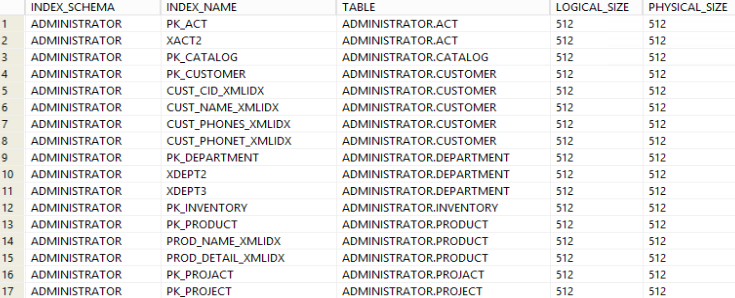## List idexes by their size in Db2 database

Article for:

Query below returns list of indexes in a database with space they use ordered from the ones using most.

## Query

``````select indschema as index_schema,
indname as index_name,
tabschema || '.' || tabname as table,
index_object_l_size as logical_size,
index_object_p_size as physical_size
where indschema not like 'SYS%'
order by physical_size,
table;
``````

## Columns

• index_schema - schema name
• index_name - index name
• table - table name on which index is defined
• logical_size - logical space used by index. The logical size is the amount of space that the table or data partition knows about.
• physical_size - physical space used by index

## Rows

• One row represents one index in a database
• Scope of rows: all indexes in a database
• Ordered by index used size from largest to smallest, table schema and table name

## Sample results